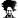# 30/360 vs Actual/360 PMT function for Amortization Table

#### RSidhu

##### Member
Hello

If you want to build amo schedule based on actual days/360 , what will your regular monthly payments (Principal + Interest) be?

I know for 30/360, it is PMT(i/12,12*loan term, original loan balance)

I dont know what would the PMT function be if it is actual/360. Wouldn't each monthly payment be different? I wonder how banks do it when they mention actual/360 in loan documents.

Thanks

#### Luke M

##### Excel Ninja
Welcome to thee forum RSidhu!I'm not sure what you mean (in financial terms).Can you elaborate and teach us?

#### Chihiro

##### Excel Ninja
Excel default for PMT is actual/360

30/360 is actually =PMT(rate/12,amortization period*12,loan amount)*12

#### RSidhu

##### Member
Excel default for PMT is actual/360

30/360 is actually =PMT(rate/12,amortization period*12,loan amount)*12
Not sure I understand. So what is the actual/360 formula then. I always wrote my PMT function as what you mentioned above (rate/12 etc.) thanks chihiro!

#### Chihiro

##### Excel Ninja
Sorry. I missed the portion where you specified monthly payment.I calculated annual payment.

Lets say for example, 6% Interest Rate, 25 year Amortization period for Loan Amount of \$1,000,000

Actual/360 monthly payment =PMT(0.06,25,1000000)/12 = \$6,518.89

30/360 monthly payment =PMT(0.06/12,25*12,1000000) = \$6,443.01
Since there's 12 month in a year.. *12/12 cancel each other out.

#### RSidhu

##### Member
Thanks Chihiro. But wouldnt the actual/360 payment be different for every month since it is "actual" no. of days for each month. If you divide it by 12, the payments become the same again. Thanks for helping btw

#### Chihiro

##### Excel Ninja
PMT is straight forward calculation on fixed loan payment schedule. And difference shows up in Rate of Interest payment to total payment.

In above example.
Actual/360: 48.87% is interest payment
30/360: 48.26% is interest payment

In practice 30/360 and Actual/360 is bit more complex, as lenders often make the monthly payment same between both (i.e. quoting lower interest rate for Actual/360). But adjust the amortization schedule to account for difference in interest. Therefore making balloon balance for Actual/360 approx 1~2% higher than 30/360.

What you may be thinking of is Actual/Actual which is most precise method of day count scheme and will account for each day of the year including leap year.

Actual/360 is obviously in favour of the lender.

http://www.margill.com/Interest-calculation-White-paper.htm

Last edited:
•NARAYANK991

#### Chihiro

##### Excel Ninja
To add to above... each day count scheme's difference is in interest accrued and interest payments. Not directly in difference of monthly payments to the lender.

#### RSidhu

##### Member
great thank you very much

#### Steve Capps

##### New Member
Many banks use an "Actual/360" formula to calculate payments, while Excel's pmt function and your financial calculator use the 30/360 formula (i.e., every month earns 30 days' interest on a 360-day year).

When banks use Actual/360, it means that interest for each day is based on the nominal rate (e.g., 6.00%) divided by 360 days. When interest accrues over 365 days, this daily rate produces a bit more than 6.00% interest income for the bank.

To calculate interest, the bank uses that daily rate based on a 360-day year, times the principal balance since the previous payment, times the actual number of days elapsed since the previous payment date.

In truth, the fully amortizing payment is a little more complex, because
the algorithm factors in the 366-day leap years during the scheduled loan term. I would guess that the algorithm calculates the total actual days in the loan term, divided by the total of the nominal 360 days in each year, times the nominal interest rate. That adjusted rate may give you the same payment if you use Excel's 30/360 formula.

The only way to know for sure is to lay out a line-by-line table of the calculation in each period. Actual days between scheduled payment dates are based on the actual days in each monthly period (31 in January, 28 in February except 29 in a leap year, etc.

What really happens during the loan is that payments are not usually posted on Saturday or Sunday, so the actual loan amortization gets skewed to the following Monday or post-holiday Tuesday. Fun, huh? And, of course, the bank earns more interest on that, too.